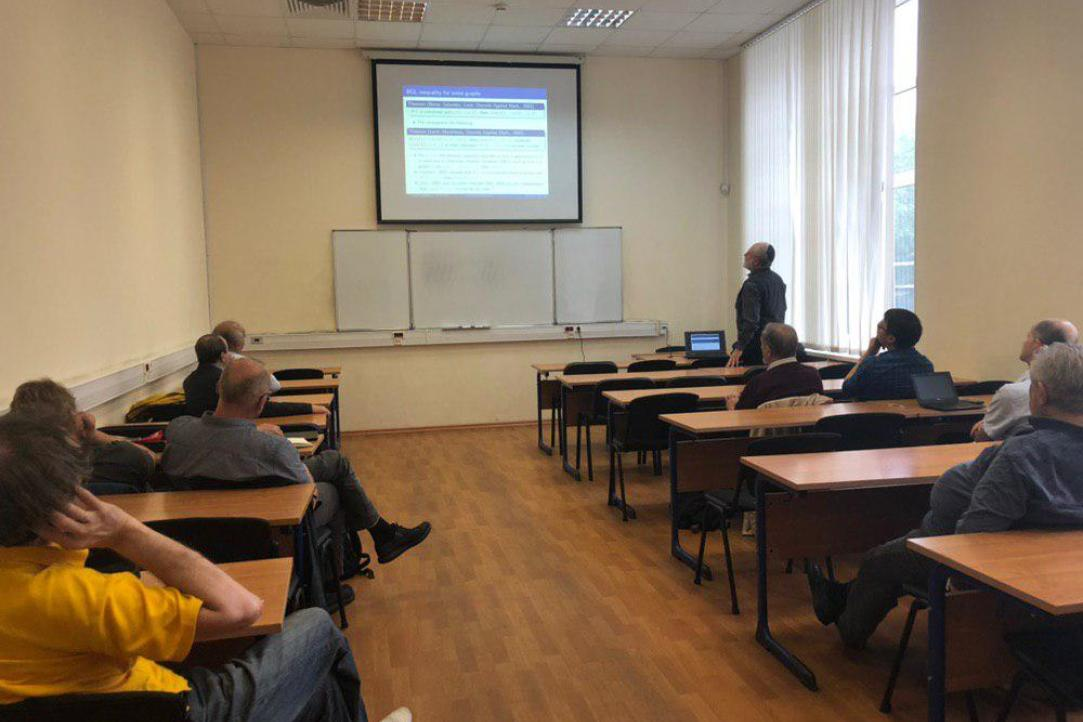• A
• A
• A
• ABC
• ABC
• ABC
• А
• А
• А
• А
• А
Regular version of the site

# Lecture by Vadim Levit on "Critical and Maximum Independent Sets Revisited"

On Wednesday, July 17 the all-Russian seminar "Mathematical methods of decision analysis in economics, finance and politics" was held. Professor Vadim Levit gave a lecture on "Critical and Maximum Independent Sets Revisited"Abstract

Let G be a simple graph with vertex set  V(G). A set S⊆V(G) is independent if no two vertices from S are adjacent, and by Ind(G) we mean the family of all independent sets of G. The number d(X)= |X|−|N(X)| is the difference of X⊆V(G), and a set A∈Ind(G) is critical if d(A)=max{d(I):I∈Ind(G)}. Let us recall the following definitions:

• core(G)=⋂{S:S is a maximum independent set},
• corona(G)=⋃{S:S is a maximum independent set},
• ker(G)=⋂{S:S is a critical independent set},
• nucleus(G)=⋂{S:S is a maximum critical independent set},
• diadem(G)=⋃{S:S is a (maximum) critical independent set}.

In this paper we focus on interconnections between ker, core, corona, nucleus, and diadem.